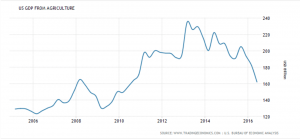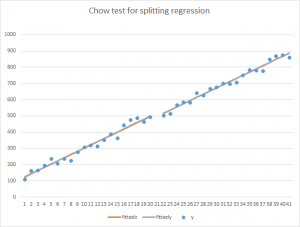6
Oct 17

## Significance level and power of test

In this post we discuss several interrelated concepts: null and alternative hypotheses, type I and type II errors and their probabilities. Review the definitions of a sample space and elementary events and that of a conditional probability.

## Type I and Type II errors

Regarding the true state of nature we assume two mutually exclusive possibilities: the null hypothesis (like the suspect is guilty) and alternative hypothesis (the suspect is innocent). It's up to us what to call the null and what to call the alternative. However, the statistical procedures are not symmetric: it's easier to measure the probability of rejecting the null when it is true than other involved probabilities. This is why what is desirable to prove is usually designated as the alternative.

Usually in books you can see the following table.

 Decision taken Fail to reject null Reject null State of nature Null is true Correct decision Type I error Null is false Type II error Correct decision

This table is not good enough because there is no link to probabilities. The next video does fill in the blanks.

## Significance level and power of test

The conclusion from the video is that$\frac{P(T\bigcap R)}{P(T)}=P(R|T)=P\text{(Type I error)=significance level}$$\frac{P(F\bigcap R)}{P(F)}=P(R|F)=P\text{(Correctly rejecting false null)=Power}$
17
Dec 16

## Testing for structural changes: a topic suitable for AP Stats

Testing for structural changes: a topic suitable for AP Stats

### Problem statement

Economic data are volatile but sometimes changes in them look more permanent than transitory.Figure 1. US GDP from agriculture. Source: http://www.tradingeconomics.com/united-states/gdp-from-agriculture

Figure 1 shows fluctuations of US GDP from agriculture. There have been ups and downs throughout the period of 2005-2016 but overall the trend has been up until 2013 and down since then. We want an objective, statistical confirmation of the fact that in 2013 the change was structural, substantial rather than a random fluctuation.

### Chow test steps

1. Divide the observed sample in two parts, A and B, at the point where you suspect the structural change (or break) has occurred. Run three regressions: one for A, another for B and the third one for the whole sample (pooled regression). Get residual sums of squares from each of them, denoted$RSS_A$$RSS_B$ and$RSS_p$, respectively.
2. Let$n_A$ and$n_B$ be the numbers of observations in the two subsamples and suppose there are$k$ coefficients in your regression (for Figure 1, we would regress GDP on a time variable, so the number of coefficients would be 2, including the intercept). The Chow test statistic is defined by$F_{k,n_A+n_B-2k}=\frac{(RSS_p-RSS_A-RSS_B)/k}{(RSS_A+RSS_B)/(n_A+n_B-2k)}.$

This statistic is distributed as$F$ with$k,n_A+n_B-2k$ degrees of freedom. The null hypothesis is that the coefficients are the same for the two subsamples and the alternative is that they are not. If the statistic is larger than the critical value at your chosen level of significance, splitting the sample in two is beneficial (better describes the data). If the statistic is not larger than the critical value, the pooled regression better describes the data.Figure 2. Splitting is better (there is a structural change)

In Figure 2, the gray lines are the fitted lines for the two subsamples. They fit the data much better than the orange line (the fitted line for the whole sample).Figure 3. Pooling is better

In Figure 3, pooling is better because the intercept and slope are about the same and pooling amounts to increasing the sample size.

23
Sep 16

## The pearls of AP Statistics 30

### Where do the confidence interval and margin of error come from?

They say: A confidence interval is an interval containing the most believable values for a parameter.
The probability that this method produces an interval that contains the parameter is called the confidence level. This is a number chosen to be close to 1, most commonly 0.95... The key is the sampling distribution of the point estimate. This distribution tells us the probability that the point estimate will fall within any certain distance of the parameter (Agresti and Franklin, p.352)... The margin of error measures how accurate the point estimate is likely to be in estimating a parameter. It is a multiple of the standard deviation of the sampling distribution of the estimate, such as 1.96 x (standard deviation) when the sampling distribution is a normal distribution (p.353)

I say: Confidence intervals, invented by Jerzy Neyman, were an important contribution to the statistical science. The logic behind them is substantial. Some math is better to hide from students but not in this case. The authors keep in mind complex notions involving math and try to deliver them verbally. Instead of hoping that students will recreate mentally those notions, why not give them directly?

### Motivation

I ask my students what kind of information they would prefer:

a) I predict the price$S$ of Apple stock to be $114 tomorrow or b) Tomorrow the price of Apple stock is expected to stay within$1 distance from \$114 with probability 95%, that is$P(113.

Everybody says statement b) is better. A follow-up question: Do you want the probability in statement b) to be high or low? Unanimous answer: High. A series of definitions follows.

An interval$(a,b)$ containing the values of a random variable$S$ with high probability

(1)$P(a

is called a confidence interval. The value$p$ which controls probability is called a confidence level and the number$\alpha=1-p$ is called a level of significance. The interpretation of$\alpha$ is that$P(S\ falls\ outside\ of\ (a,b))=\alpha$ as follows from (1).

### How to find a confidence interval

We want the confidence level to be close to 1 and the significance level to be close to zero. In applications, we choose them and we need to find the interval$(a,b)$ from equation (1).

Step 1. Consider the standard normal. (1) becomes$P(a. Note that usually it is impossible to find two unknowns from one equation. Therefore we look for a symmetric interval, in which case we have to solve

(2)$P(-a

for$a$. The solution$a=z_{cr}$ is called a critical value corresponding to the confidence level$p$ or significance level$\alpha=1-p$. It is impossible to find it by hand, that's why people use statistical tables. In Mathematica, the critical value is given by$z_{cr}$=Max[NormalCI[0, 1, ConfidenceLevel -> p]].

Geometrically, it is obvious that, as the confidence level approaches 1, the critical value goes to infinity, see the video or download the Mathematica file.

Step 2. In case of a general normal variable, plug its z-score in (2):

(3)$P(-z_{cr}<\frac{X-\mu}{\sigma}

The event$-z_{cr}<\frac{X-\mu}{\sigma} is the same as$-z_{cr}\sigma which is the same as$\mu-z_{cr}\sigma. Hence, their probabilities are the same:

(4)$P(-z_{cr}<\frac{X-\mu}{\sigma}

We have found the confidence interval$(\mu-z_{cr}\sigma,\mu+z_{cr}\sigma)$ for a normal variable. This explains where the margin of error$z_{cr}\sigma$ comes from.

Step 3. In case of a random variable which is not necessarily normal we can use the central limit theorem. z-scores of sample means$z=\frac{\bar{X}-E\bar{X}}{\sigma(\bar{X})}$, for example, approach the standard normal. Instead of (3) we have an approximation$P(-z_{cr}<\frac{\bar{X}-E\bar{X}}{\sigma(\bar{X})}

Then instead of (4) we get$P(E\bar{X}-z_{cr}\sigma(\bar{X})<\bar{X}

In my classes, I insist that logically interconnected facts should be given in one place. To consolidate this information, I give my students the case of one-sided intervals as an exercise.

14
Mar 16

## What is a p value?

What is a p value? The definition that is easier to apply

This blog discusses how tricky the notion of a p-value is. It states the technical definition of a p-value — the probability of getting results at least as extreme as the ones you observed, given that the null hypothesis is correct. Stated like this, I don't understand it either. The definition from Wikipedia is not much clearer: In frequentist statistics, the p-value is a function of the observed sample results (a test statistic) relative to a statistical model, which measures how extreme the observation is. The p-value is defined as the probability of obtaining a result equal to or "more extreme" than what was actually observed, assuming that the model is true.

Below I give the definition I prefer, hoping that it's easier to apply. This discussion requires the knowledge of the null and alternative hypotheses and of the significance level. It also presumes availability of some test statistic (t statistic for simplicity).

Suppose we want to test the null hypothesis$H_0:\ \beta=0$ against a symmetric alternative$H_a:\ \beta\neq0$. Given a small number$\alpha\in(0,1)$ (which is called a significance level in this context) and an estimator$\hat{\beta}$ of the parameter$\beta$, consider the probability$f(\alpha)=P(|\hat{\beta}|>\alpha)$. Note that when$\alpha$ decreases to$0$, the value$f(\alpha)$ increases. In my book, I use the following definition: the p-value is the smallest significance level at which the null hypothesis can still be rejected. With this definition, it is easier to understand that (a) the null is rejected at any$\alpha\geq p$-value and (b) for any$\alpha-value the decision is ”Fail to reject the null”.

Remarks. Statistical procedures for hypotheses testing are not perfect. In particular, there is no symmetry between the null and alternative hypotheses. The fact that their choice is up to the researcher makes the test subjective. The choice of the significance level is subjective, as is the choice of the model and test statistic. Users with limited statistical knowledge tend to overestimate the power of statistics.